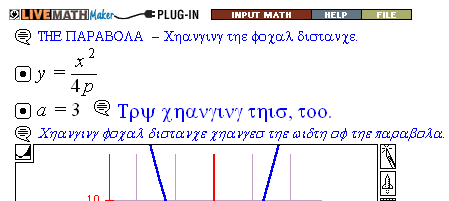Search IntMath
Close

450+ Math Lessons written by Math Professors and Teachers

5 Million+ Students Helped Each Year

1200+ Articles Written by Math Educators and Enthusiasts

Simplifying and Teaching Math for Over 23 Years

# Greek fonts in LiveMath

By Murray Bourne, 20 Nov 2006

## Update [May 2014]

I used to use LiveMath extensively on IntMath, but the product was not kept up to date, and there was no support, so I dropped it entirely.

A shame, because it was quite a good product in its time.

The Interactive Mathematics site (used to) use LiveMath throughout so that users can learn by changing variables, animating graphs, solving algebra problems and multiplying matrices, etc.

I generally like(d) the LiveMath plugin, but at times it behave(d) weirdly. It replace(d) English words with Greek characters, like this:It is an intermittent problem and when it happens, it happens in Firefox, IE and Opera. I find if I check again after a few days, it is okay, suggesting it is session-dependent.

Have you experienced this?

Be the first to comment below.

### Comment Preview

HTML: You can use simple tags like <b>, <a href="...">, etc.

To enter math, you can can either:

1. Use simple calculator-like input in the following format (surround your math in backticks, or qq on tablet or phone):
a^2 = sqrt(b^2 + c^2)
(See more on ASCIIMath syntax); or
2. Use simple LaTeX in the following format. Surround your math with $$ and $$.
$$\int g dx = \sqrt{\frac{a}{b}}$$
(This is standard simple LaTeX.)

NOTE: You can mix both types of math entry in your comment.